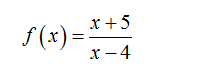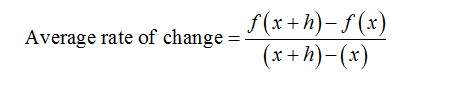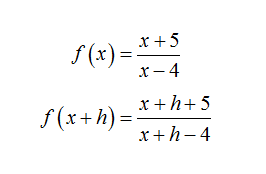# Given the function f(x) = x+5/x-4 Find the average rate of chnage of f on [x,x+h]

Question
5 views

Given the function f(x) = x+5/x-4

Find the average rate of chnage of f on [x,x+h]

check_circle

Step 1

Given function:Step 2

The average rate of chnage of function over interval [x,x+h]:Step 3

Now,...

### Want to see the full answer?

See Solution

#### Want to see this answer and more?

Solutions are written by subject experts who are available 24/7. Questions are typically answered within 1 hour.*

See Solution
*Response times may vary by subject and question.
Tagged in

### Algebra